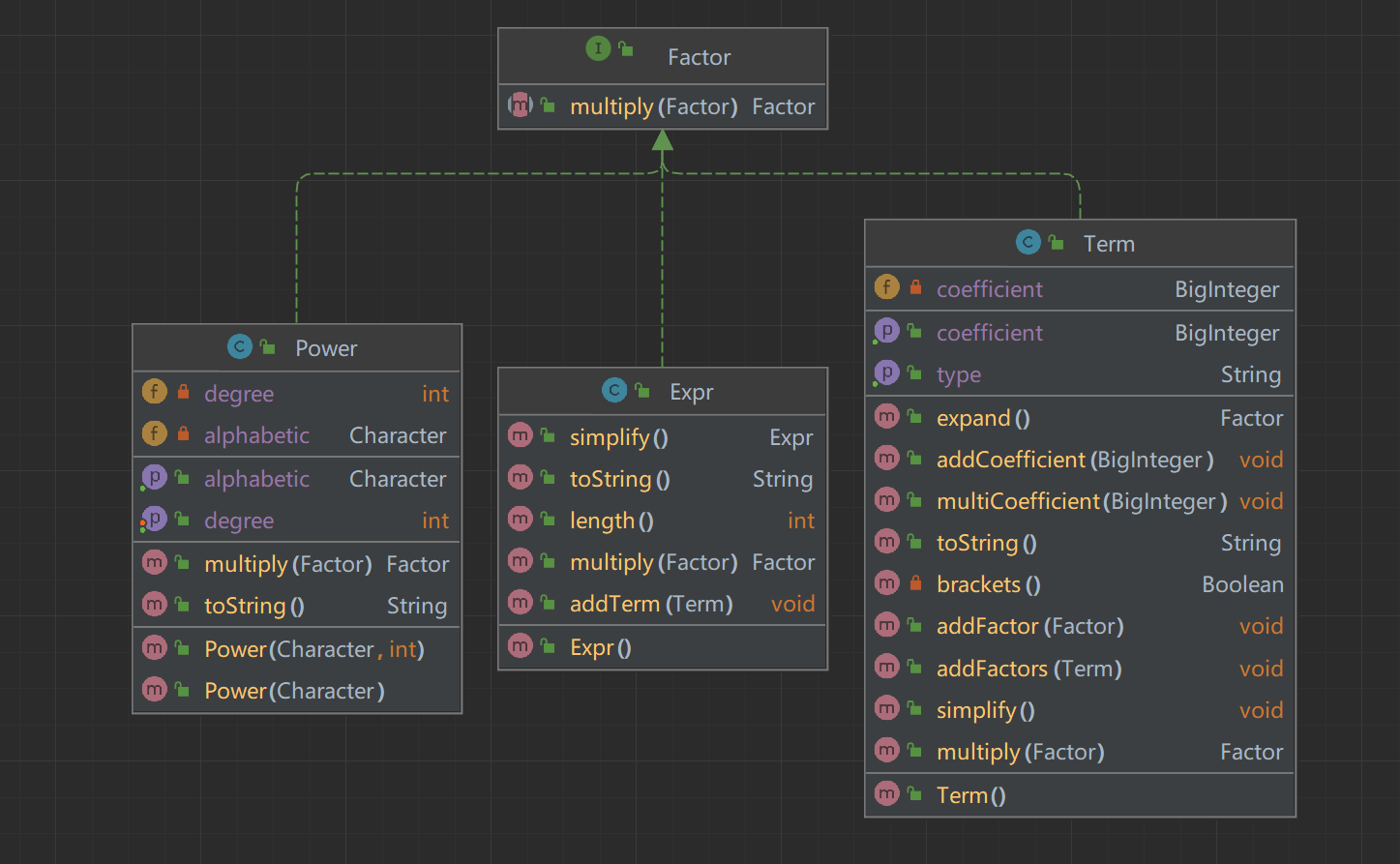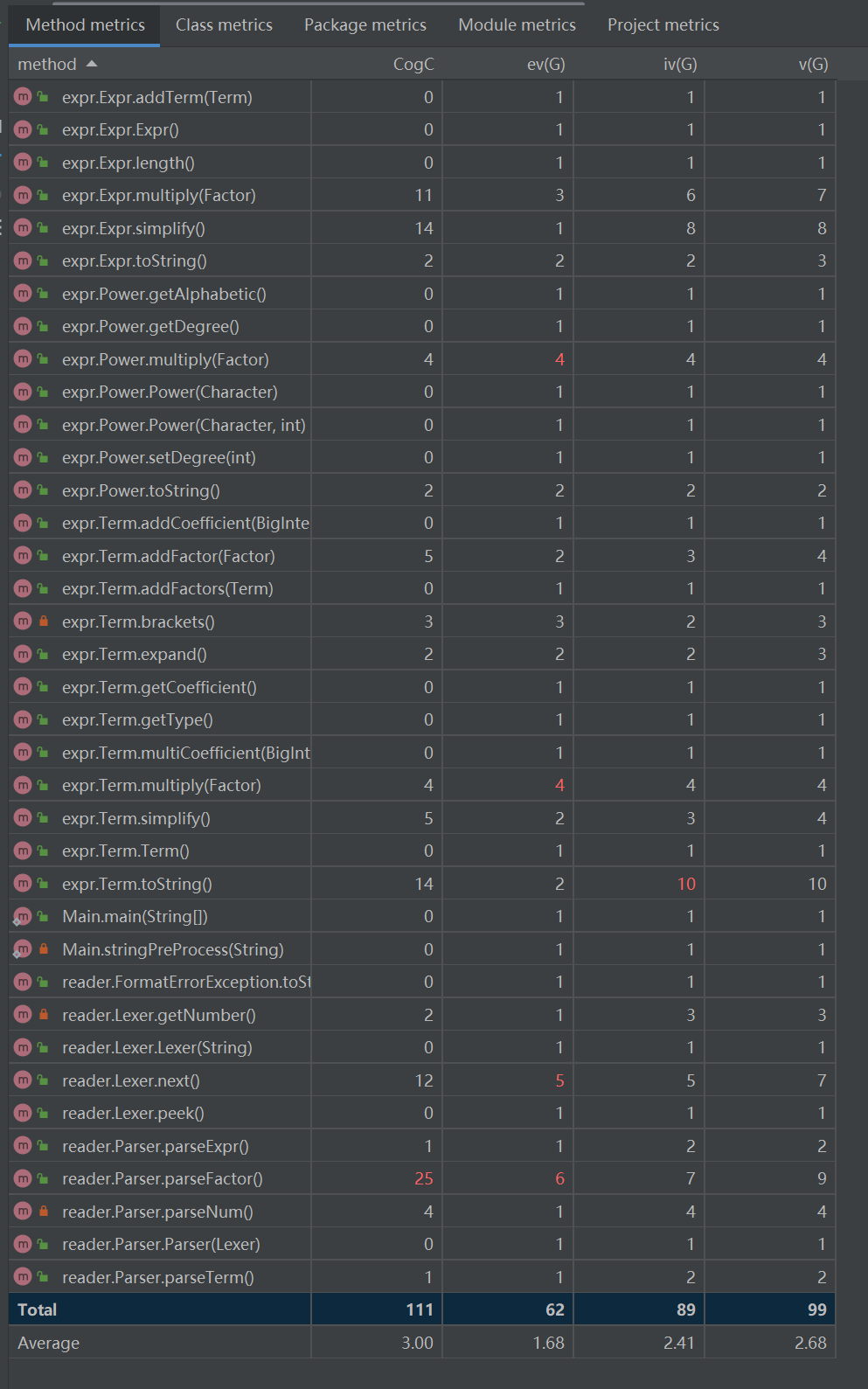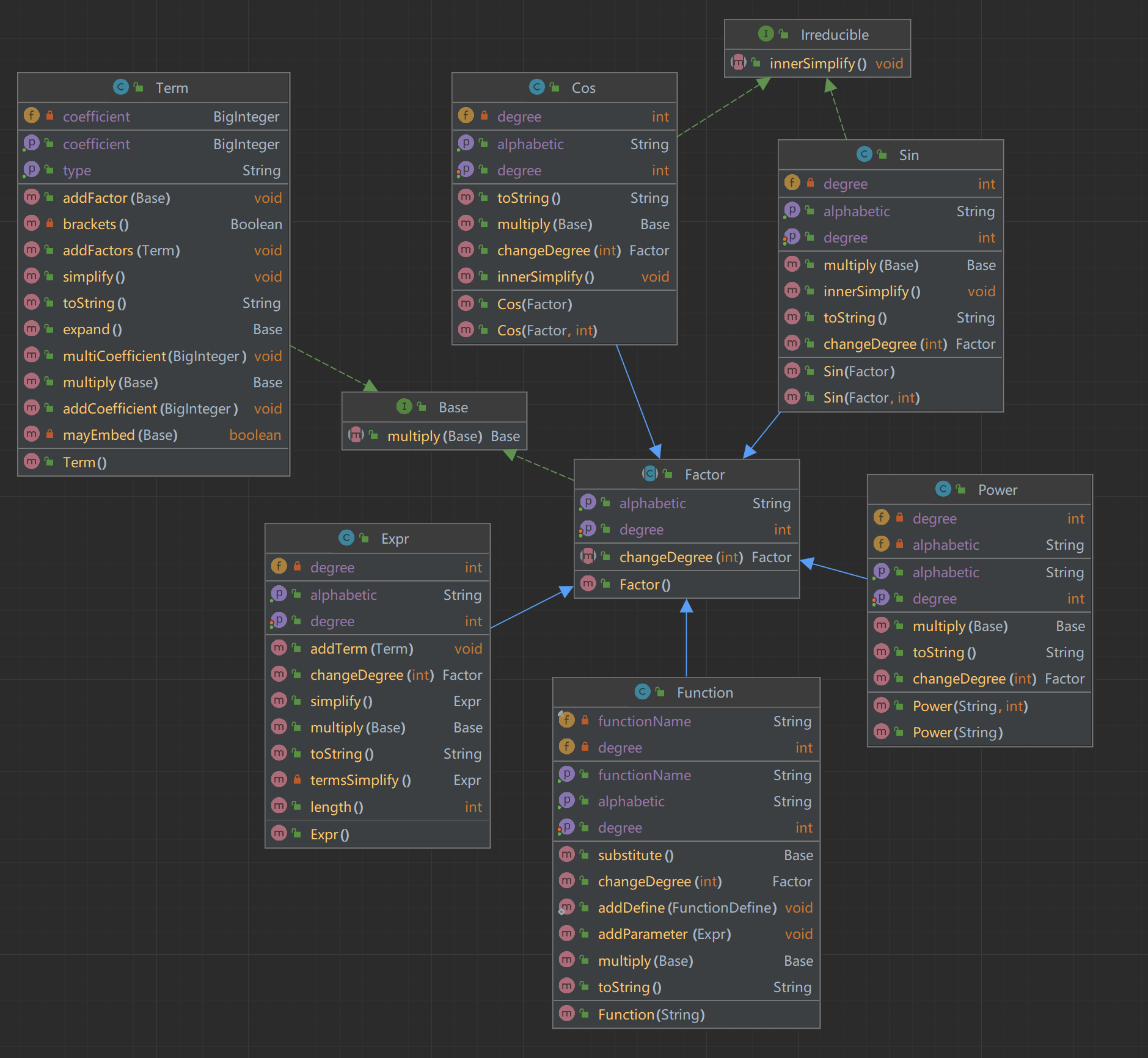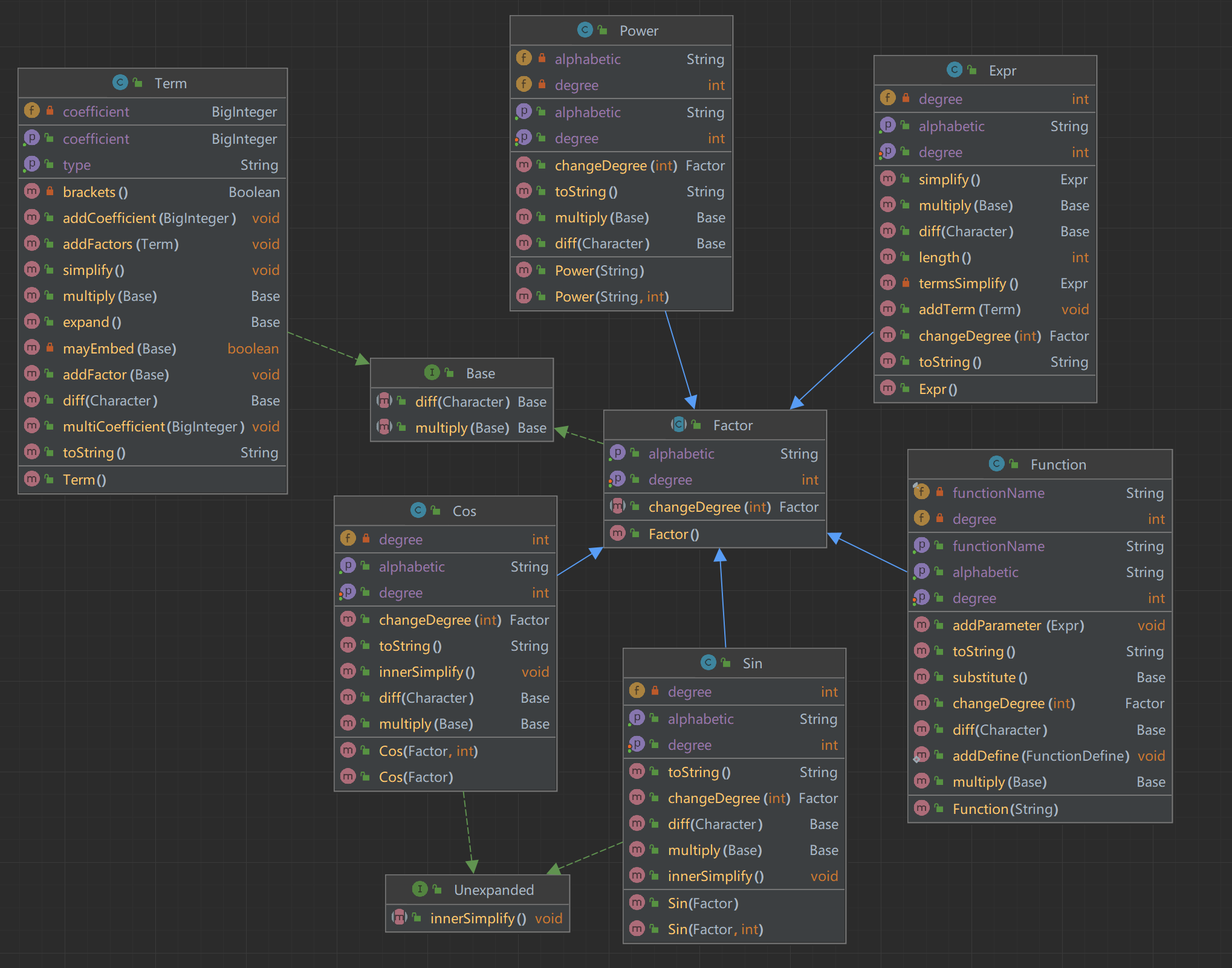# Homework 1

## 类图## OO 度量## BUG 分析

``````    private static String stringPreProcess(String s) {
return s.replaceAll("[ \r\t]", "")
.replaceAll("-\\+", "-")
.replaceAll("\\+-", "-")
.replaceAll("--", "+")
.replaceAll("\\+\\+", "+")
.replaceAll("\\*\\*\\+", "**");
}``````

``````    private static String stringPreProcess(String s) {
return s.replaceAll("[ \r\t]", "")
.replaceAll("-\\+", "-")
.replaceAll("\\+-", "-")
.replaceAll("--", "+")
.replaceAll("\\+\\+", "+")
.replaceAll("-\\+", "-")
.replaceAll("\\+-", "-")
.replaceAll("--", "+")
.replaceAll("\\+\\+", "+")
.replaceAll("\\*\\*\\+", "**");
}``````

## BUG 测试

``````def generate(recursion=1):
ans = ''
expr_len = random.randint(1, 6)
for i in range(expr_len):
item_len = random.randint(1, 4)
ans += str(random.randint(-5, +5))
for j in range(item_len):
ans += '*'
if recursion > 0 and random.randint(0, 4) == 3:
ans += '('
ans += generate(recursion - 1)
ans += ')'
if random.randint(0, 4) == 3:
ans += '**'
if random.randint(0, 1) == 0:
ans += '+'
ans += str(random.randint(0, 4))
else:
if random.randint(0, 4) != 3:
ans += value_map[random.randint(0, 2)]
if random.randint(0, 4) == 3:
if random.randint(0, 4) == 3:
ans += '**'
if random.randint(0, 1) == 0:
ans += '+'
ans += str(random.randint(0, 4))
else:
ans += str(random.randint(-10, 10))

if i != expr_len - 1:
if random.randint(0, 1) == 1:
ans += '+'
else:
ans += '-'
return ans``````

# Homework 2

## 基本架构

Base 接口：这个接口被所有的表达式相关类实现，它只有一个方法是 multiply。

Irreducible 接口：这个接口被 Sin，Cos 等类实现，这个接口中包含一个方法 innerSimplify。他的设计中实现这个接口的类是拥有内部表达式，并且这个内部表达式的化简不会对外部产生任何影响的。举个例子来说，sin((2*x)) 永远不会被化简为 2*sin(x)*cos(x)。 这个接口以后还可以 implements 到 exp、ln 等类上。

Factor 类：这是一个虚类，所有的因子都继承于这个类。

## 类图# Homework 3

## 类图# 心得体会

...全文
19 回复 打赏 收藏 举报BUAA OO Unit1 单元总结
BUAA OO Unit1 总结BUAA OO UNIT1总结多项式求导系列——OO Unit1分析总结[OO] Unit1 Summary 表达式求导系列
[OO] Unit1 SummaryOO第一次博客作业
OO Unit1 总结 ​ 前三次作业以多项式求导为核心，每次作业在上一次基础上加大难度，在完成这些作业以后，我对于面向对象的设计方法、思想迭代开发的模式有了一定的理解，以下是对第一单元三次作业的分析和一些心得体会。 第一次作业 task：实现简单多项式的求导，因子仅为带符号整数与幂函数。 类图 设计思想：由于第一次因子较为简单，我采用了HashMap类型对单个项进行建模，设计一个多项式类来完成...